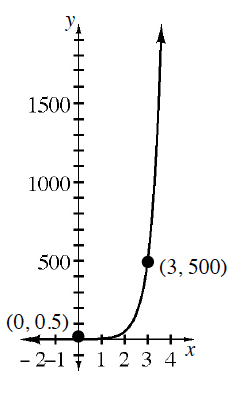### Home > INT1 > Chapter 10 > Lesson 10.1.2 > Problem10-30

10-30.

Use the graph at right to write a possible equation in $y = ab^{x}$ form.

The graph shows two specific points that the graph passes through.
$(0, 0.5)$ and $(3, 500)$

Review the Math Notes box in section 8.2.2